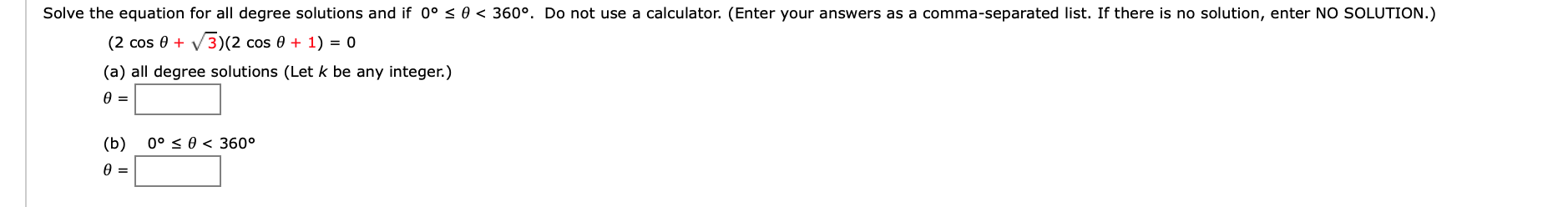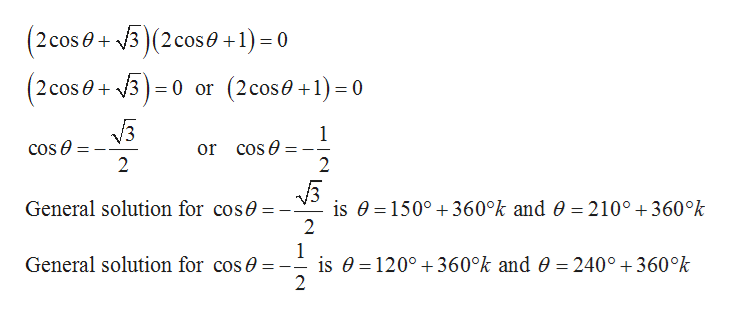# Solve the equation for all degree solutions and if 0° < 0 < 360°. Do not use a calculator. (Enter your answers as a comma-separated list. If there is no solution, enter No SOLUTION.)3)(2 cos 0(2 cos 01)oV(a) all degree solutions (Let k be any integer.)Ө —(b)0° S 0< 360°

Question
1 viewshelp_outlineImage TranscriptioncloseSolve the equation for all degree solutions and if 0° < 0 < 360°. Do not use a calculator. (Enter your answers as a comma-separated list. If there is no solution, enter No SOLUTION.) 3)(2 cos 0 (2 cos 0 1) o V (a) all degree solutions (Let k be any integer.) Ө — (b) 0° S 0< 360° fullscreen
check_circle

Step 1

(a)

Given:

Step 2

Find the value of the e...help_outlineImage Transcriptionclose(2 cos0+3 (2 cose +1) = 0 2 cose3 0 or (2cos0+1)0 /3 cos e or cos e 2 2 General solution for cose is 0 1500 360°k and 0 = 210° +360°k 2 1 is 0 120° 360°k and 0 = 240°360°k 2 General solution for cos fullscreen

### Want to see the full answer?

See Solution

#### Want to see this answer and more?

Solutions are written by subject experts who are available 24/7. Questions are typically answered within 1 hour.*

See Solution
*Response times may vary by subject and question.
Tagged in

### Trigonometry